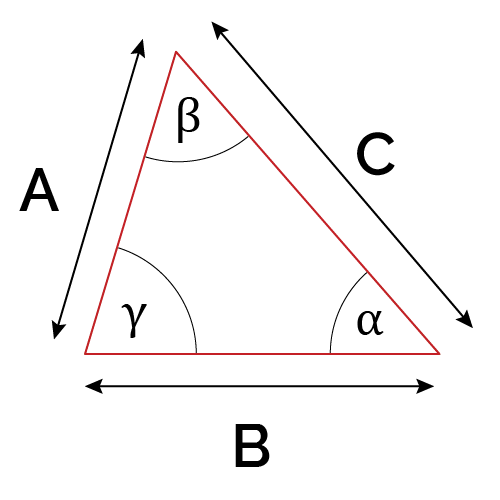# Solve Similar Triangles Calculator

Created by Luis Fernando
Reviewed by Dominika Miszewska, MD, PhD candidate and Steven Wooding
Last updated: Jul 23, 2022

Our similar triangles calculator solves for any x unknown value within two similar triangles. Keep reading to know how to solve similar triangles for any x unknown value with this calculator.

## Application of this calculator for similar triangles: Solve for an x unknown height

Suppose somebody wants to know the height of the tree in the following image:

Luckily, it's a sunny day and that person places themselves 16 ft away from the tree, which is a distance at which the shadow height equals that person's height. For this reason, the angle formed by the tree's shadow and the person's shadow is the same.

As you can see in the image, both shadows and the ground form two similar triangles. If the length of the person's height equals 8 ft, what is the height of the tree? You can quickly know the answer using this solve similar triangles calculator:

1. In the solve similar triangles calculator, the triangle formed by the person and its shadow is the first triangle.
2. As the person forms a 90° degree angle with the floor, and we know two of the triangle sides, select "angle and 2 sides" as the given information.
3. In "Angle selection," select "γ" as the known angle.
4. Input γ = 90 degrees, a = 5 ft, b = 8 ft, and for the second triangle, input the corresponding B = 24 ft value.
5. That's it! The tree height should be 15 ft, as given by A. That's how you use this calculator in similar triangles to solve for an x unknown value.

## FAQ

### How do I solve similar triangles?

To solve similar triangles:

1. Select two corresponding segments of both triangles.
2. Divide the first triangle segments by the second triangle segments. That is the similarity scale.
3. To know an unknown value of the first triangle, multiply it by the similarity scale.
4. For a value of the second triangle, divide by the similarity scale.

### How can you use similar triangles to solve real-world problems?

In real-world problems, you can use similar triangles to determine the size of objects you cannot measure. The most common example is calculating the unknown height of an object you can't measure, using the shadow formed by that object and a second reference object.

### How to solve an A = 48, a = 32, b = 27 triangle?

To solve a triangle of A = 48, a = 32, b = 27:

1. Use the formula a/sin(A) = b/sin(B)
2. Solve for B:
• B = arcsin[(b/a)sin(A)]
• B = arcsin[(27/32)sin(48°)]
• B = 38.83°
3. Check if another angle B can exist.
4. Subtract B = 38.83° from 180° to find the possible second angle.
B₂ = 180° - 38.83° = 141.17°
B₂ + A = 141.17° + 48° = 189.17°
6. As the sum is higher than 180°, the second angle is not valid, and there's only one possible B angle: B = 38.83°.
Luis Fernando
Help
1) Input information about the first triangle. 2) One of the sides of the second triangle. The calculator will find the remaining dimensions of the second triangle.
First triangle
Given
angle and 2 sides
Angle selection
αα
deg
a
in
b
in
c
in
β
deg
γ
deg
Second triangle
A
in
B
in
C
inPeople also viewed…

### Black Friday

How to get best deals on Black Friday? The struggle is real, let us help you with this Black Friday calculator!

### Car heat

The hot car calculator shows you how fast the interior of a car heats up during a summers day.

### Matrix multiplication

The matrix multiplication calculator is your little helper in finding the product of two matrices up to 3x3 in size.

### Triangle scale factor

Use this triangle scale factor calculator to find the scale factor between two similar triangles.